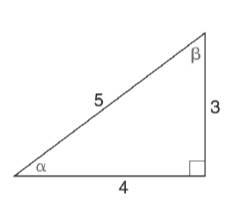Chapter 11.3, Problem 1E### Elementary Geometry for College St...

6th Edition
Daniel C. Alexander + 1 other
ISBN: 9781285195698

#### Solutions

Chapter
Section### Elementary Geometry for College St...

6th Edition
Daniel C. Alexander + 1 other
ISBN: 9781285195698
Textbook Problem
86 views

# In Exercises 1 to 4, find tan α and tan β for each triangle.To determine

To find:

The values of tanα and tanβ for the triangle.

Explanation

Given:

The triangle is shown below.

Definition used:

In a right triangle, the tangent ratio for an acute angle is the ratio oppositeadjacent.

Calculation:

First let us calculate tanα

### Still sussing out bartleby?

Check out a sample textbook solution.

See a sample solution

#### The Solution to Your Study Problems

Bartleby provides explanations to thousands of textbook problems written by our experts, many with advanced degrees!

Get Started

#### In Exercises 14, find the values of x that satisfy the inequality (inequalities). 4. 2x2 50

Applied Calculus for the Managerial, Life, and Social Sciences: A Brief Approach

#### The fourth term of {an} defined by a1 = 3, and for n = 1, 2, 3, … is:

Study Guide for Stewart's Single Variable Calculus: Early Transcendentals, 8th

#### The graph of x = cos t, y = sin2 t is:

Study Guide for Stewart's Multivariable Calculus, 8th